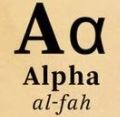D

#### In the game Scrabble, there are a total of 100 tiles. Of the 100 tiles, 42 tiles have the vowels A, E, I, O, and U printed on them, 56 tiles have the consonants printed on them, and 2 tiles are left blank.

64 viewed last edited 2 years agoAnonymous
0

) 1)1a. If tiles are selected at random, what is the probability that the first tile drawn from the pile of 100 tiles is a vowel?

b. If tiles drawn are not replaced, what is the probability that the first two tiles selected are both vowels?

c. Event A is drawing a vowel, event B is drawing a consonant, and event C is drawing a blank tile.A1  means a vowel is drawn on the first selection, B2 means a consonant is drawn on the second selection, and  C2 means a blank tile is drawn on the second selection. Tiles are selected at random and without replacement.

1) P( A1 and B2)

2) P( A1 and B2)

3) P(B1 and C2)Anuj Gupta
0

a. Since 42 titles have a vowel in them and there are a total of 100 tiles The probability that a vowel is drawn from the total number of tiles is \frac{42}{100} = 0.42 .

b. The probability that the first two tiles selected are both vowels is $\frac[1}{42} \cdot \frac[1}{42} = 0.174$.

c.

1) P( A1 and B2) is the probability of selecting both a vowel and a consonant. We know that the probability of selecting a vowel is \frac{42}{100} and since there are 56 tiles which have consonants and since there are 99 tiles left after selecting one tile, the probability of selecting a consonant from the 99 tiles is \frac{56}{99}. So, the probability of both A1 and B2 happening is \frac{42}{100} \cdot \frac{56}{99} \approx 0.238.

2) P(A1 and C2) = \frac{42}{100} \cdot \frac{2}{99} \approx 0.008

3) P(B1 and C2) = \frac{56}{100} \cdot \frac{2}{99} \approx 0.011